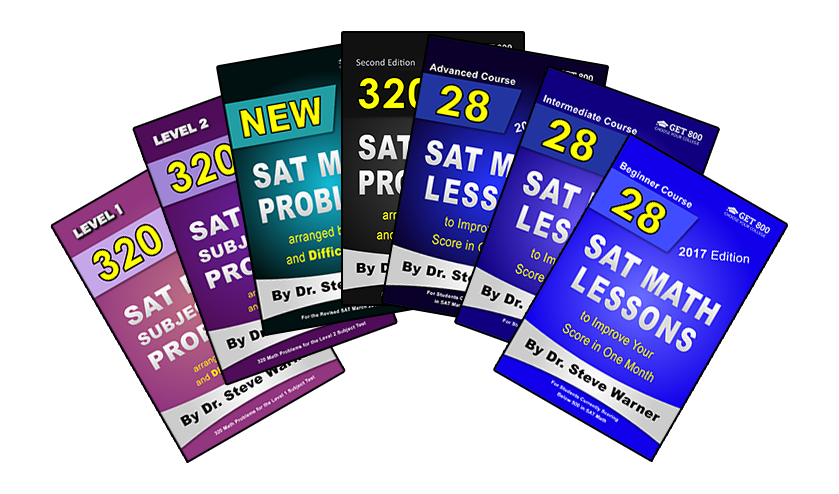# Blog

##### SAT Level 5 Heart of Algebra Problem with Solution##### SAT Level 5 Heart of Algebra Problem with Solution

Today I would like to give you a difficult Heart of Algebra problem with a solution. Additional methods of solution will be provided in the next few days.

Level 5 Heart of Algebra

A worker earns \$12 per hour for the first 40 hours he works in any given week, and \$18 per hour for each hour above 40 that he works each week. If the worker saves 75% of his earnings each week, what is the least number of hours he must work in a week to save at least \$441 for the week?

A) 6
B) 8
C) 46
D) 47

* Informal solution:
If \$441 represents 75% of the worker’s earnings, then the worker’s total earnings is 441/0.75 = \$588.

For the first 40 hours, the worker earns 12⋅40=480 dollars. So, the remaining amount that the worker needs to earn is 588-480=108 dollars. Therefore, the number of additional hours above 40 that the worker will work is 108/18 = 6.

The total number of hours that the worker must work is therefore 40 + 6 = 46, choice C.

Notes: (1) We change a percent to a decimal by moving the decimal point to the left 2 places. The number 75 has a “hidden” decimal point at the end of the number (75 = 75. or 75.0). When we move this decimal point to the left two places we get .75 or 0.75.

(2) We can find the worker’s total earnings formally as follows:

We are given that 441 is 75% of the worker’s total earnings. So, we have 441 = 0.75T, where T is the worker’s total earnings. We divide each side of this equation by 0.75 to get T = 441/0.75 = 588.

(3) Be careful that you do not accidentally choose 6 as the answer. 6 is the number of hours above 40 that the worker must work. The question is asking for the total number of hours, which is 40 + 6.

If you’re preparing for the SAT, you may want to check out the Get 800 collection of SAT math books.##### COLLeGE PLANnING CHECKLIST FOR EVERY YEAR OF HIGH SCHOOL

Download this FREE checklist from InGenius Prep for action points on how to build your college candidacy throughout high school.

Download this FREE checklist from InGenius Prep for action points on how to build your college candidacy throughout high school.

Less
##### Common App Guide Ebook

Download this FREE eBook from InGenius Prep and learn how to take

advantage of every Common App section.

Download this FREE eBook from InGenius Prep and learn how to take  advantage of every Common App section.

Less